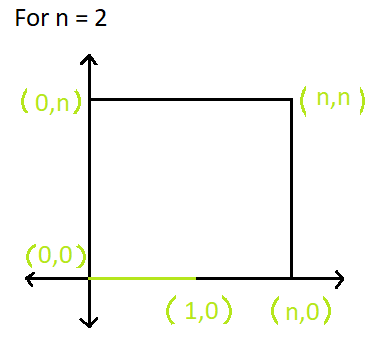# Shortest path on a Square

• Last Updated : 31 May, 2021

Given side of a square n and two points (x1, y1) and (x2, y2) on the boundaries of the given square. The task is to find the shortest path through the square sides between these two points where the corner coordinates of the square are (0, 0), (n, 0), (0, n), and (n, n).

Examples:

Attention reader! All those who say programming isn't for kids, just haven't met the right mentors yet. Join the  Demo Class for First Step to Coding Coursespecifically designed for students of class 8 to 12.

The students will get to learn more about the world of programming in these free classes which will definitely help them in making a wise career choice in the future.

Input: n = 2, x1 = 0, y1 = 0, x2 = 1, y2 = 0
Output:Input: n = 26, x1 = 21, y1 = 0, x2 = 26, y2 = 14
Output: 19

Approach:

• If both the x and y coordinates of a point is greater than the other and the points are not on opposite sides of square then the shortest distance will be abs(x2 – x1) + abs(y2 – y1).
• Else, the shortest distance will be equal to min((x1 + y1 + x2 + y2), (4 * n) – (x1 + y1 + x2 + y2))

Below is the implementation of the above approach:

## C++

 `// C++ implementation of the approach``#include ``using` `namespace` `std;` `// Function to return the length``// of the minimum path between``// two points on a square of given side``int` `minPath(``int` `n, ``int` `x1, ``int` `y1, ``int` `x2, ``int` `y2)``{` `    ``// If both of the x and y coordinates``    ``// of one point is greater than the other``    ``if` `((x1 <= x2 && y1 <= y2) || (x1 >= x2 && y1 >= y2))``    ``{``        ``// If the points are not on opposite sides``        ``if` `(!(``abs``(y2 - y1) == n || ``abs``(x2 - x1) == n))``            ``return` `(``abs``(x1 - x2) + ``abs``(y1 - y2));``    ``}``    ``return` `min(x1 + x2 + y1 + y2, (4 * n) - (x1 + x2 + y1 + y2));``}` `// Driver code``int` `main()``{``    ``// Side of the square``    ``int` `n = 4;``    ``int` `x1 = 2, y1 = 0, x2 = 3, y2 = 4;``    ``cout << minPath(n, x1, y1, x2, y2);` `    ``return` `0;``}` `// improved by Sonal Agrawal`

## Java

 `// Java implementation of the approach``class` `GFG{` `// Function to return the length``// of the minimum path between``// two points on a square of given side``static` `int` `minPath(``int` `n, ``int` `x1, ``int` `y1,``                          ``int` `x2, ``int` `y2)``{``    ` `    ``// If both of the x and y coordinates``    ``// of one point is greater than the other``    ``if` `((x1 <= x2 && y1 <= y2) ||``        ``(x1 >= x2 && y1 >= y2))``    ``{``        ` `        ``// If the points are not on opposite sides``        ``if` `(!(Math.abs(y2 - y1) == n ||``              ``Math.abs(x2 - x1) == n))``            ``return` `(Math.abs(x1 - x2) +``                    ``Math.abs(y1 - y2));``    ``}``    ``return` `Math.min(x1 + x2 + y1 + y2, (``4` `* n) -``                   ``(x1 + x2 + y1 + y2));``}` `// Driver code``public` `static` `void` `main(String[] args)``{``    ` `    ``// Side of the square``    ``int` `n = ``4``;``    ``int` `x1 = ``2``, y1 = ``0``, x2 = ``3``, y2 = ``4``;``    ` `    ``System.out.println(minPath(n, x1, y1, x2, y2));``}``}` `// This code is contributed by sanjeev2552`

## Python3

 `# Python3 implementation of above approach` `# Function to return the length of the``# minimum path between two points``# on a square of given side``def` `minPath(n, x1, y1, x2, y2):` `    ``# If both of the x and y coordinates``    ``# of one point is greater than the other``    ``if` `(((x1 <``=` `x2 ``and` `y1 <``=` `y2) ``or``        ``(x1 >``=` `x2 ``and` `y1 >``=` `y2)) ``and``        ``not` `(``abs``(y2 ``-` `y1) ``=``=` `n ``or``             ``abs``(x2 ``-` `x1) ``=``=` `n)):``        ``return` `(``abs``(x1 ``-` `x2) ``+` `abs``(y1 ``-` `y2));` `    ``return` `min``(x1 ``+` `x2 ``+` `y1 ``+` `y2, (``4` `*` `n) ``-``              ``(x1 ``+` `x2 ``+` `y1 ``+` `y2));` `# Driver code` `# side of the square``n ``=` `4``; x1 ``=` `2``; y1 ``=` `0``x2 ``=` `3``; y2 ``=` `4``print``(minPath(n, x1, y1, x2, y2))` `# This code is contributed``# by Shashank_Sharma`

## C#

 `// C# implementation of the approach``using` `System;` `class` `GFG``{``    ` `// Function to return the length``// of the minimum path between``// two points on a square of given side``static` `int` `minPath(``int` `n, ``int` `x1, ``int` `y1,``                        ``int` `x2, ``int` `y2)``{` `    ``// If both of the x and y coordinates``    ``// of one point is greater than the other``    ``if` `((x1 <= x2 && y1 <= y2) || (x1 >= x2 && y1 >= y2))``    ``{``        ``// If the points are not on opposite sides``        ``if` `(!(Math.Abs(y2 - y1) == n || Math.Abs(x2 - x1) == n))``            ``return` `(Math.Abs(x1 - x2) + Math.Abs(y1 - y2));``    ``}``    ``return` `Math.Min(x1 + x2 + y1 + y2, (4 * n) - (x1 + x2 + y1 + y2));``}` `// Driver code``public` `static` `void` `Main()``{``    ``// Side of the square``    ``int` `n = 4;``    ``int` `x1 = 2, y1 = 0, x2 = 3, y2 = 4;``    ``Console.Write(minPath(n, x1, y1, x2, y2));``}``}` `// This code is contributed``// by Akanksha Rai`

## PHP

 `= ``\$x2` `&& ``\$y1` `>= ``\$y2``))``    ``{``        ``// If the points are not on opposite sides``        ``if` `(!(``abs``(``\$y2` `- ``\$y1``) == ``\$n` `|| ``abs``(``\$x2` `- ``\$x1``) == ``\$n``))``            ``return` `(``abs``(``\$x1` `- ``\$x2``) + ``abs``(``\$y1` `- ``\$y2``));``    ``}``    ``return` `min(``\$x1` `+ ``\$x2` `+ ``\$y1` `+ ``\$y2``, (4 * ``\$n``) - (``\$x1` `+ ``\$x2` `+ ``\$y1` `+ ``\$y2``));``    ` `}` `// Driver code` `// Side of the square``\$n` `= 4;``\$x1` `= 2 ;``\$y1` `= 0 ;``\$x2` `= 3 ;``\$y2` `= 4 ;``echo` `minPath(``\$n``, ``\$x1``, ``\$y1``, ``\$x2``, ``\$y2``);``    ` `// This code is contributed by Ryuga``?>`

## Javascript

 ``
Output
`7`

My Personal Notes arrow_drop_up